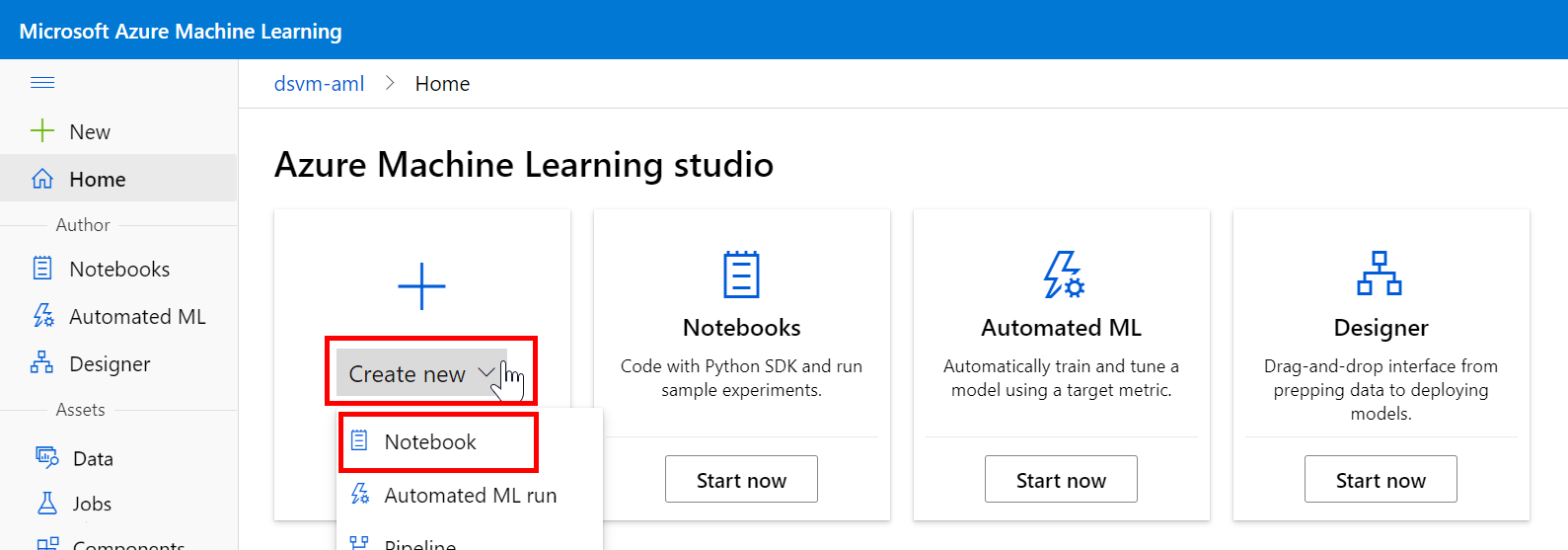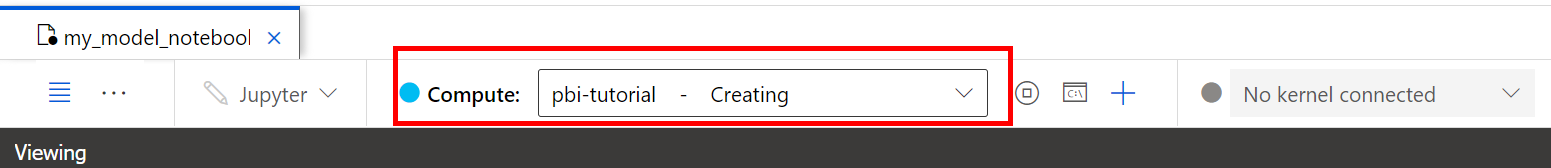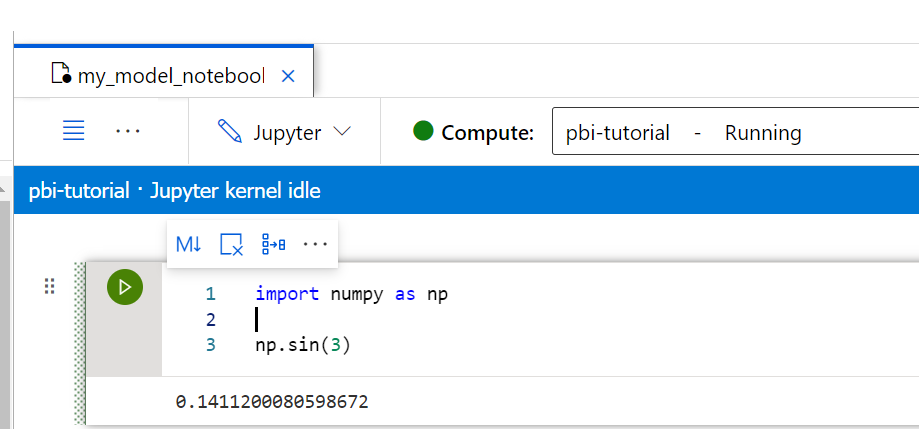# 教學課程：Power BI 整合 - 使用 Jupyter Notebook 建立預測模型 (第 1 部分，共 2 部分)

• 建立 Jupyter Notebook。
• 建立 Azure Machine Learning 計算執行個體。
• 使用 scikit-learn 訓練迴歸模型。
• 寫入評分指令碼來定義輸入和輸出，以便與 Microsoft Power BI 輕鬆整合。
• 將模型部署到即時評分端點。

## 先決條件

• Azure 訂用帳戶。 如果您沒有訂用帳戶，可以使用免費試用
• Azure Machine Learning 工作區。 若您尚未擁有工作區，請參閱建立工作區資源
• Python 語言和機器學習工作流程的簡介知識。

## 建立筆記本和計算

Azure Machine Learning Studio 首頁中，選取 [新建] > [筆記本]：1. 為您的筆記本命名 (例如 my_model_notebook)。
2. 檔案類型變更為筆記本
3. 選取 [建立]。1. 選擇 CPU 虛擬機器大小。 在本教學課程中，您可以選擇具有 2 個核心和 14 GB RAM 的 Standard_D11_v2
2. 選取 [下一步] 。
3. 設定頁面上，提供有效的計算名稱。 有效的字元為大寫和小寫字母、數字和連字號 (-)。
4. 選取 [建立]。``````import numpy as np

np.sin(3)
``````## 使用 scikit-learn 建置模型

### 匯入資料

``````from azureml.opendatasets import Diabetes

diabetes = Diabetes.get_tabular_dataset()
X = diabetes.drop_columns("Y")
y = diabetes.keep_columns("Y")
X_df = X.to_pandas_dataframe()
y_df = y.to_pandas_dataframe()
X_df.info()
``````

`X_df` Pandas 資料框架包含 10 個基準輸入變數。 這些變數包含年齡、性別、身體質量指數、平均血壓和六種血清測量值。 `y_df` Pandas 資料框架是目標變數， 並包含在基準後一年疾病進展的量化量值。 資料框架包含 442 筆記錄。

### 將模型定型

``````import joblib
from sklearn.linear_model import Ridge

model = Ridge().fit(X_df,y_df)
joblib.dump(model, 'sklearn_regression_model.pkl')
``````

### 註冊模型

``````import sklearn

from azureml.core import Workspace
from azureml.core import Model
from azureml.core.resource_configuration import ResourceConfiguration

ws = Workspace.from_config()

model = Model.register(workspace=ws,
model_name='my-sklearn-model',                # Name of the registered model in your workspace.
model_path='./sklearn_regression_model.pkl',  # Local file to upload and register as a model.
model_framework=Model.Framework.SCIKITLEARN,  # Framework used to create the model.
model_framework_version=sklearn.__version__,  # Version of scikit-learn used to create the model.
sample_input_dataset=X,
sample_output_dataset=y,
resource_configuration=ResourceConfiguration(cpu=2, memory_in_gb=4),
description='Ridge regression model to predict diabetes progression.',
tags={'area': 'diabetes', 'type': 'regression'})

print('Name:', model.name)
print('Version:', model.version)
``````## 定義評分指令碼

• 當服務啟動時，就會執行 `init()` 函式。 此函式會載入模型 (模型會從模型登錄中自動下載) 並將其還原序列化。
• 當服務的呼叫包含需要計分的輸入資料時，就會執行 `run(data)` 函式。

``````%%writefile score.py

import json
import pickle
import numpy as np
import pandas as pd
import os
import joblib
from azureml.core.model import Model

from inference_schema.schema_decorators import input_schema, output_schema
from inference_schema.parameter_types.numpy_parameter_type import NumpyParameterType
from inference_schema.parameter_types.pandas_parameter_type import PandasParameterType

def init():
global model
# Replace filename if needed.
path = os.getenv('AZUREML_MODEL_DIR')
model_path = os.path.join(path, 'sklearn_regression_model.pkl')
# Deserialize the model file back into a sklearn model.

input_sample = pd.DataFrame(data=[{
"AGE": 5,
"SEX": 2,
"BMI": 3.1,
"BP": 3.1,
"S1": 3.1,
"S2": 3.1,
"S3": 3.1,
"S4": 3.1,
"S5": 3.1,
"S6": 3.1
}])

# This is an integer type sample. Use the data type that reflects the expected result.
output_sample = np.array()

# To indicate that we support a variable length of data input,
# set enforce_shape=False
@input_schema('data', PandasParameterType(input_sample))
@output_schema(NumpyParameterType(output_sample))
def run(data):
try:
print("input_data....")
print(data.columns)
print(type(data))
result = model.predict(data)
print("result.....")
print(result)
# You can return any data type, as long as it can be serialized by JSON.
return result.tolist()
except Exception as e:
error = str(e)
return error
``````

## 定義自訂環境

``````from azureml.core.model import InferenceConfig
from azureml.core import Environment

environment = Environment('my-sklearn-environment')
'azureml-defaults',
'inference-schema[numpy-support]',
'joblib',
'numpy',
'pandas',
'scikit-learn=={}'.format(sklearn.__version__)
])

inference_config = InferenceConfig(entry_script='./score.py',environment=environment)
``````

## 部署模型

``````service_name = 'my-diabetes-model'

service = Model.deploy(ws, service_name, [model], inference_config, overwrite=True)
service.wait_for_deployment(show_output=True)
``````

``````Tips: You can try get_logs(): https://aka.ms/debugimage#dockerlog or local deployment: https://aka.ms/debugimage#debug-locally to debug if deployment takes longer than 10 minutes.
Running......................................................................................
Succeeded
ACI service creation operation finished, operation "Succeeded"
````````````import json